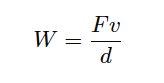Articles

# What is a Weigh Feeder ?

A special type flow meter suited for powdered or granular solids is the weigh feeder. One of the most common weigh feeder designs consists of a conveyor belt with a section supported by rollers coupled to one or more load cells, such that a fixed length of the belt is continuously weighed:

The load cell measures the weight of a fixed-length belt section, yielding a figure of material weight per linear distance on the belt. A tachometer (speed sensor) measures the speed of the belt. The product of these two variables is the mass flow rate of solid material “through” the weigh feeder:Where,
W = Mass flow rate (e.g. pounds per second)
F = Force of gravity acting on the weighed belt section (e.g. pounds)
v = Belt speed (e.g. feet per second)
d = Length of weighed belt section (e.g. feet)

A small weigh feeder (about two feet in length) is shown in the following photograph, the weigh feeder being used to feed powdered soda ash into water at a municipal filtration plant to neutralize pH:

In the middle of the belt’s span (hidden from view) is a set of rollers supporting the weight of the belt and of the soda ash piled on the belt. This load cell array provides a measurement of pounds material per foot of belt length (lb/ft).

As you can see in this next picture, the soda ash powder simply falls off the far end of the conveyor belt, into the water below:

The speed sensor measures belt speed in feet per minute (ft/min). This measurement, when multiplied by the pounds-per-foot measurement sensed by the load cells, translates into a mass flow rate (W) in units of pounds per minute (lb/min). A simple unit conversion (×60) expresses the mass flow rate in units of pounds per hour (lb/h).

A photograph of this weigh feeder’s display screen shows these variables:

Note that the belt loading of 1.209 lb/ft and the belt speed of 0.62 feet per minute do not exactly equate to the displayed mass flow rate of 43.7 lb/h.

The reason for this discrepancy is that the camera’s snapshot of the weigh feeder display screen happened to capture an image where the values were not simultaneous. Weigh feeders often exhibit fluctuations in belt loading during normal operation, leading to fluctuations in calculated mass flow rate.

Sometimes these fluctuations in measured and calculated variables do not coincide on the display screen, given the latency inherent to the mass flow calculation (delaying the flow rate value until after the belt loading has been measured and displayed).